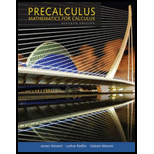Chapter 7.1, Problem 82E### Precalculus: Mathematics for Calcu...

7th Edition
James Stewart + 2 others
ISBN: 9781305071759

#### Solutions

Chapter
Section### Precalculus: Mathematics for Calcu...

7th Edition
James Stewart + 2 others
ISBN: 9781305071759
Textbook Problem

# Proving Identities Verify the identity.82. sec v − tan v = 1 sec v + tan v

To determine

To show: The given identity, that is secυtanυ=1secυ+tanυ .

Explanation

Proof:

Simplify the left hand side of the given expression by multiplying the numerator and denominator by secυ+tanυ as follows:

secυtanυ=(secυtanυ)(secυ+tanυ)secυ+tanυ=sec2υtan2υsecυ+tanυ

### Still sussing out bartleby?

Check out a sample textbook solution.

See a sample solution

#### The Solution to Your Study Problems

Bartleby provides explanations to thousands of textbook problems written by our experts, many with advanced degrees!

Get Started

## Additional Math Solutions

#### In Exercises 1520, simplify the expression. 15. 4(x2+y)3x2+y

Applied Calculus for the Managerial, Life, and Social Sciences: A Brief Approach

#### Given: mB=75mDCE=50 Find: mD and mDEF

Elementary Geometry For College Students, 7e

#### Read each scale:

Elementary Technical Mathematics

#### Determine the infinite limit. limxcotx

Single Variable Calculus: Early Transcendentals

#### It does not exist.

Study Guide for Stewart's Multivariable Calculus, 8th